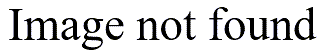# Interactions in logistic regression

## 2004-04-08

Someone asked me how to compute interactions in binary logistic regression. You need to be careful, since interactions are tricky to interpret. I need to update my pages to offer a detailed description of what an interaction is and how to interpret it properly.

I did find the time to run a simple example of interaction using the Titanic mortality data set. In that data set, women had much better survival probabilities (this was during an era when people believed in the phrase “Women and children first”). The effect, however, is not as strong in 3rd class passengers as it is in first and second class passengers.When you calculate a separate odds ratio for each passenger class, you get a value of 30 for first class, 42 for second class, and 4.6 for third class.This disparity is the classic example of interaction–the effect of one variable becomes stronger or weaker depending on the level of the other variable.

When you fit a binary logistic model with an interaction, the estimates look like this:The last passenger class (third class) becomes the reference level. The odds ratio for sex, 4.6, is the odds ratio within the third passenger class. The odds ratio for the interaction between pclass(1) and sex is 6.6, which is shows how much larger the odds ratio would be in first class compared to third class. It is literally a ratio of odds ratios (30/4.6). The odds ratio for the interaction between pclass(2) and sex is 9.3, which again shows how much larger the odds ratio is in second class compared to third class (9.3 = 43/4.6).

If you detect a significant interaction in your data set, the simplest thing to do is to estimate odds ratios separately for one of the two factors involved in the interaction. For example, someone wrote in asking about an interaction between gender (male/female) and alcohol use (yes/no) in a logistic regression model predicting dating violence. The simplest thing is to look at the odds ratio relating drinking and dating violence first for males only and then for females only. That seems like the logical choice, but you could also look at the odds ratio for gender and dating violence, first for the drinkers and then for the teetotalers.

You can find an earlier version of this page on my original website. Interactions in logistic regression